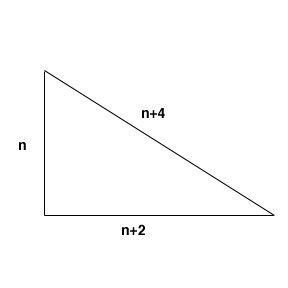SEARCH HOMEMath Central Quandaries & QueriesBrad, a parent: I am having a problem coming up with a formula for my son's eighth grade math problem. We have found the answer by guess and check but have a mental block on the equation. Any help would be appreciated. The problem is: A right triangle has sides whose lengths in feet are consecutive even intergers. Determine the length of each side. ThanksEach consecutive even integer is separate by 2 so the sides would be n, n+2 and n+4. Since the hypotenuse is the longest side, I know that will be n+4.We can use the Pythagorean Theorem to solve for n. So then
n2+(n+2)2=(n+4)2
n2+n2+4n+4=n2+8n+16
2n2+4n+4=n2+8n+16
n2-4n+12=0
(n-6)(n+2)=0
n=6 or n=-2
Since the side of the triangle cannot be negative, n=6. So the length of the sides are 6, 8 & 10.

Hope this helps. If your son has not learned factoring or polynomial multiplication in school yet, guess and check might be the best option.

JaniceMath Central is supported by the University of Regina and The Pacific Institute for the Mathematical Sciences.